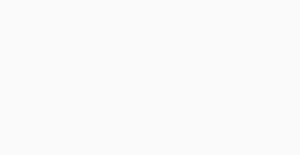#Quants Questions : Data Interpretation Set 13

Hello Aspirants. Welcome to Online Quantitative Aptitude section in AffairsCloud.com. Here we are creating question sample From Data Intrepretation that is important for IBPS,SBI exam,SSC exam, Railways Exam,FCI exam  and other competitive exams. We have included Some questions that are repeatedly asked in exams !!

I.Study the information carefully to answer the given questions
In a college out of 600 students, boys and girls are in the ratio 8: 7. Each students went to either  physics lab or chemical lab or both, 40% of the boys attend only physics lab, total 20% children went  to both the labs out of which 25% are boys, 45% of the girls went to only chemistry lab.

1. Total how many boys went to only physics lab ?
A)175
B)158
C)156
D)134
E)None of these
B)158
Explanation :8:7 = 320(boys):280(girls)
20% both = 20*600/100 = 120
B – (phy ) = 40*320/100 = 128
B – (both) = 25*120/100 = 30
G – (chem) = 45*280/100 = 126
Total no of boys(phy) = 128+30 = 158

2. How many girls went to only physics lab ?
A)62
B)46
C)64
D)60
E) None of these
C) 64
Explanation :No of girls (phy) = (280-126 – 90) = 64

3. Total number of students went to physics lab is what percentage of the total number of students in the college
A)22%
B)32%
C)33%
D)36%
E) None of these
B)32%
Explanation :
Total no of students went to only phy = 128+64 = 192
% = 192*100/600 = 32%

4. What is the total number of girls went to chemistry lab ?
A)216
B)210
C)267
D)261
E) None of these
A)216
Explanation :Total no of Girls(chem) = 126+90 = 216

5. What is the total number of students went to only chemistry lab ?
A)244
B)248
C)284
D)288
E) None of these
D)288
Explanation :Total no of students(chem) = (320-128-30)B + 126G = 162 + 126 = 288

II. Study the information carefully to answer the given questions
No of 5 types of books sold and returned(due to missing of pages) by a publisher over the years(data in 10000)

 Book B1 B2 B3 B4 B5 Year Sold Return Sold Return Sold Return Sold Return Sold Return 2001 22 4 52 5 17 2.5 82 7 62 6 2002 37 5 47 4 22 2.55 77 6 60 6 2003 17 2.5 42 4.5 19 2.75 60 4 64 5.5 2004 27 2.25 44 4.3 27 3.5 67 5 42 3.5 2005 32 3.5 50 4.5 32 4 70 5 47 4 2006 29 3.5 43 4.1 28 2.75 74 2.5 52 4.5
1. In the case of B2 type of books, in which year was the ratio of return to sold highest among the five years ?
A)2001
B)2002
C)2003
D)2004
E)2005
C)2003
Explanation :
2001 = 5/52 =0.0962
2002 = 4/47 = 0.0851
2003 = 4.5/42 = 0.1071
2004 =4.3/44 = 0.0977
2005 = 4.5/50 = 0.09
2006 = 4.1/43 = 0.0953

2. In the year 2002 ,  ratio of book return to sold was  below 8.5% in which type of books  ?
A)B1
B)B2
C)B3
D)B4
E)B5
D)B4
Explanation :
B1 =(5*100)/37 = 13.51%
B2 =(4*100)/47 = 8.51%
B3 =(2.55*100)/22 = 11.59%
B4 =(6*100)/77 = 7.79%
B5 =(6*100)/60 = 10%

3. In the case of B5 type of books, in which year was the return to sold rate is lowest among the 6 years ?
A)2006
B)2002
C)2003
D)2005
E)2004
E)2004
Explanation :
2001 = 6/62 = 0.0968
2002 = 6/60 = 0.10
2003 = 5.5/64 = 0.0859
2004 = 3.5/42 = 0.0833
2005 = 4/47 = 0.0851
2006 = 4.5/52 = 0.0865

4. The acceptable B5 type of books in 2003 was what percent of that of that in 2002 ?
A)108.33%
B)103.88%
C)108.44%
D)103.54%
E)None of these
A)108.33%
Explanation :
(64-5.5)*10,000 = 585000………….2003
(60-6)*10,000 = 540000……………..2002
% = (585000*100)/540000 = 108.33%

5. The number of B3 type of books returned in 2002 is approximately what % more or less than B4 type of books rejected in 2003 ?
A)36.2%
B)32.65%
C)36.25%
D)15.65%
E)None of these
C)36.25%
Explanation :
2002    = 2.55*10000 = 25500
2003    = 4*10000 = 40000
% = [(40000-25500)*100]/ 40000 = 36.25%

AffairsCloud Recommends Oliveboard Mock Test

AffairsCloud Ebook - Support Us to Grow